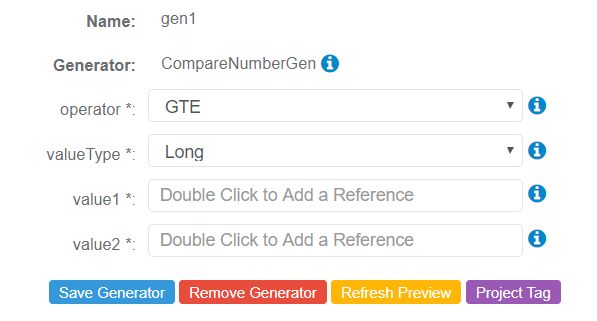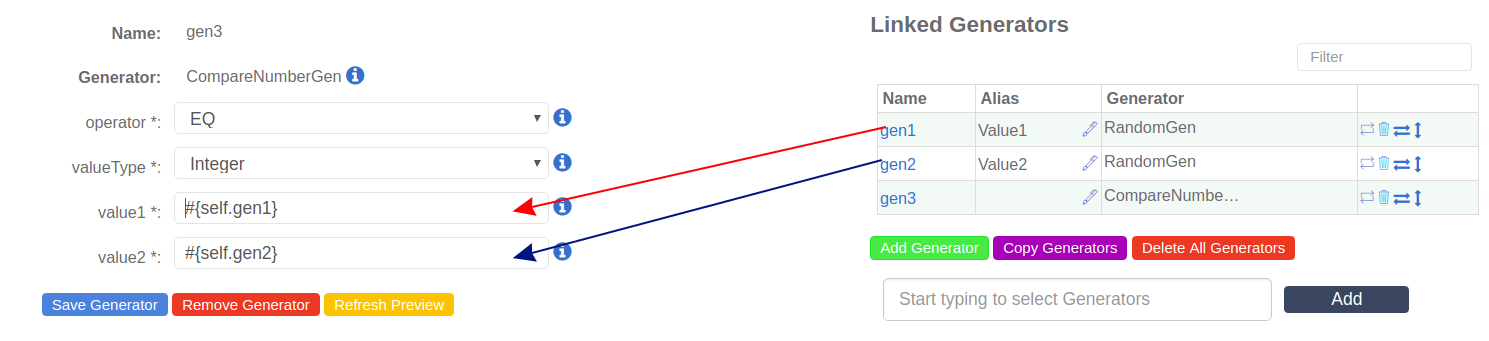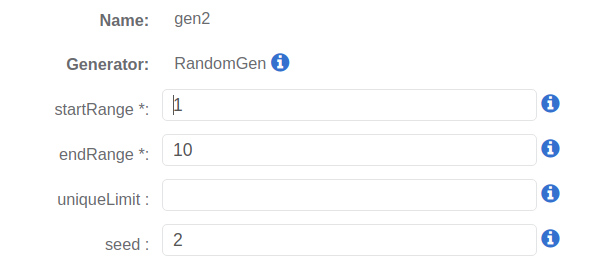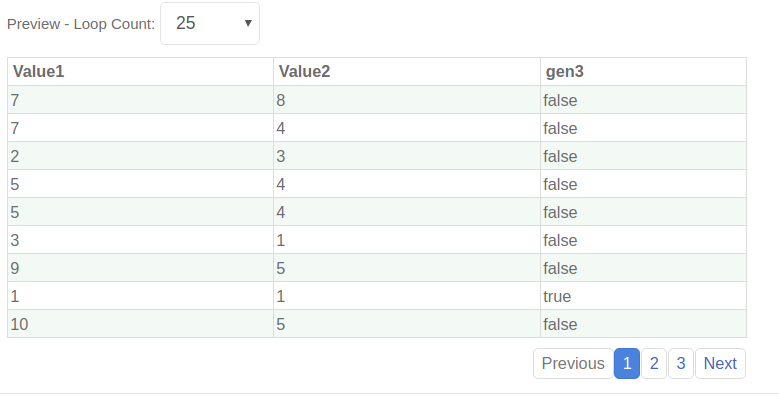# Description

The CompareNumberGen Generator compares two numbers using [EQ, NE, LT, LTE, GT or GTE] and returns a Boolean result.

## Parameters

The following parameters may be configured for the CompareNumberGen Generator. Items with an asterisk* are required.

• operator* - Defines how the two numbers will be compared to each other.

• EQ - Equal

• NE - Not Equal

• LT - Less Than

• LTE - Less Than Equal

• GR - Greater Than

• GTE - Greater Than Equal

• valueType* - Defines type of numbers to be compared.

• value1* - The left side of the comparison.

• value2* - The right side of the comparison.## Example 1: Check if number in value1 Parameter is equal to number in value2 Parameter

This example involves three linked Generators: RandomGen (Twice) and the CompareNumberGen. For this example, the following Parameters have been configured for the CompareNumberGen Generator:

• operator = EQ (Equal)
• valueType = Integer
• value1 = References RandomGen (gen1)
• value2 = References RandomGen (gen2)The configurations for each RandomGen Generator are shown below:

Value 1:Value 2:## Sample Output

gen3 = The result of the comparison if value 1 is equal to value 2.